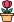엔지니어 게시판
LeetCode 솔루션 분류

# [11/20] 224. Basic Calculator

### 작성자 정보

•작성
• 작성일

• 933 조회
• 1 댓글
• 0 추천
• 0 비추천

### 본문

Hard

Given a string `s` representing a valid expression, implement a basic calculator to evaluate it, and return the result of the evaluation.

Note: You are not allowed to use any built-in function which evaluates strings as mathematical expressions, such as `eval()`.

Example 1:

```Input: s = "1 + 1"
Output: 2
```

Example 2:

```Input: s = " 2-1 + 2 "
Output: 3
```

Example 3:

```Input: s = "(1+(4+5+2)-3)+(6+8)"
Output: 23
```

Constraints:

• `1 <= s.length <= 3 * 105`
• `s` consists of digits, `'+'``'-'``'('``')'`, and `' '`.
• `s` represents a valid expression.
• `'+'` is not used as a unary operation (i.e., `"+1"` and `"+(2 + 3)"` is invalid).
• `'-'` could be used as a unary operation (i.e., `"-1"` and `"-(2 + 3)"` is valid).
• There will be no two consecutive operators in the input.
• Every number and running calculation will fit in a signed 32-bit integer.
Accepted
354,605
Submissions
841,931

댓글 1

## 학부유학생님의 댓글

• 익명
• 작성일
Runtime: 229 ms, faster than 42.49% of Python3 online submissions for Basic Calculator.
Memory Usage: 18.1 MB, less than 9.85% of Python3 online submissions for Basic Calculator.
``````class Solution:
def calculate(self, s: str) -> int:
s = list(s)
stack = []

def calc(op, num):
if op == "+":
stack.append(num)
elif op == "-":
stack.append(-num)

operators = ["+","-"]
op = "+"
num = 0

for char in s+["+"]:

if char in operators:
calc(op, num)
op = char
num = 0
elif char.isdigit():
num = num*10 + int(char)

elif char == "(":
stack.append(op)
op = "+"
num = 0

elif char == ")":
calc(op, num)
op = "+"
num = 0
temp = 0
while stack[-1] not in operators:
temp += stack.pop()

temp_op = stack.pop()
calc(temp_op, temp)

return sum(stack)``````
전체 349 / 1 페이지

• 등록일 19:01
• 등록일 17:45
• 등록일 14:27
• 등록일 14:25
• 등록일 14:23
• 등록일 14:20
• 등록일 14:17
• 등록일 14:13
• 등록일 14:12
• 등록일 14:06
• 등록일 14:03
• 등록일 14:01

• 등록일 01.31
• 등록일 01.31
• 등록일 01.31
• 등록일 01.27
• 등록일 01.27
• 등록일 01.23
• 등록일 01.23

### Stats

• 현재 접속자 84 명
• 오늘 방문자 1,679 명
• 어제 방문자 1,866 명
• 최대 방문자 2,853 명
• 전체 회원수 535 명